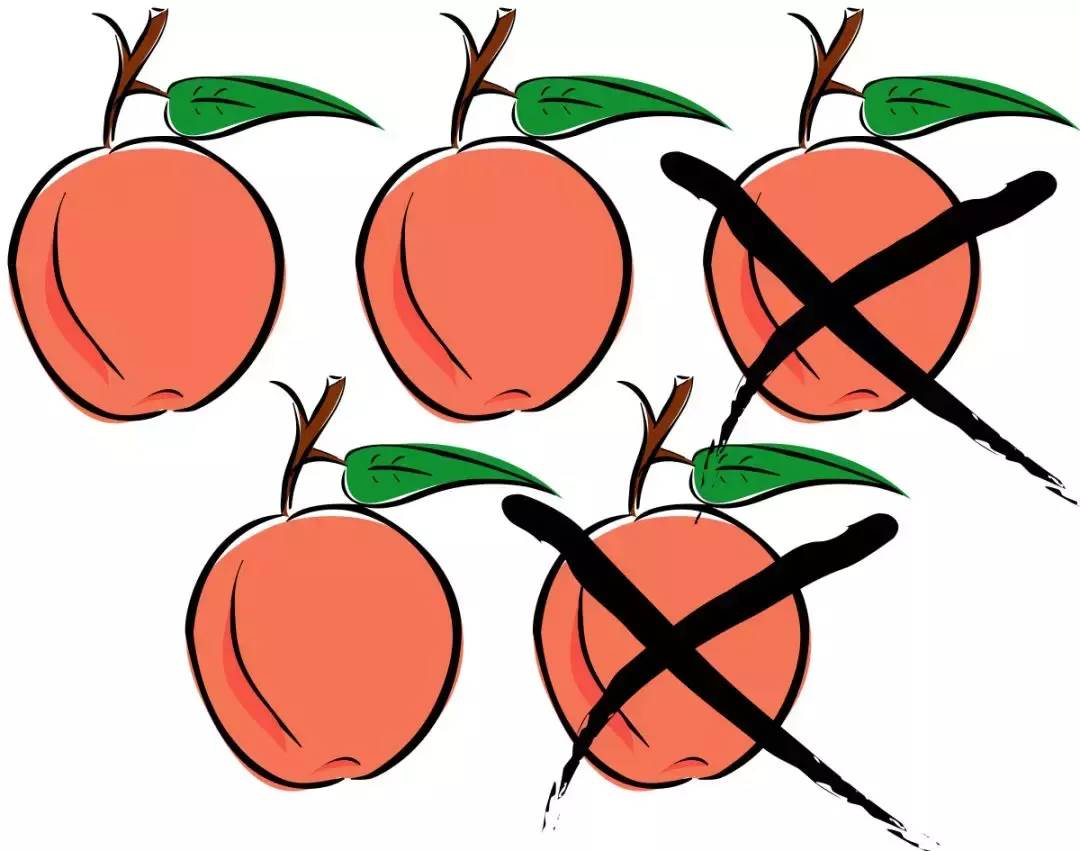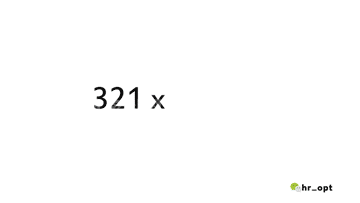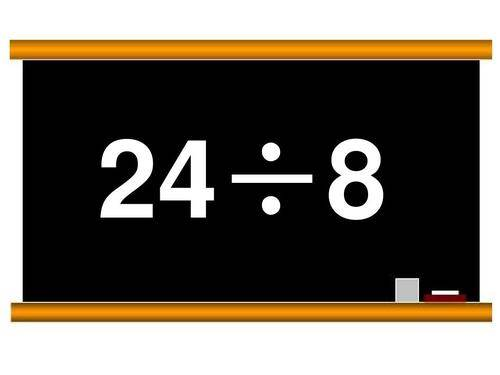# 「加，減，乘，除」的英語都不會？英語老師在盯著你

Can you help with the little boy‘s math problem？

16  take away 8 is what？1、減（ - ） take away， minus， subtract

Nine  take away six is three. （9-6=3）

Ten  subtract two is eight. （10-2=8）

noun：

take aways subtraction

-I need to do take aways.

-Can you help me with this subtraction problem？

Attention： subtraction is more than take aways.

Question：

How do you say 12-3=9 in English？

2、加Two  plus three is five. （2+3=5）

six  add two is eight. （6+2=8）

Question：

How do you say 5+5=10 in English？

3、乘以（x） times or multiply by

Four  times three is twelve （4x3=12）

Multiply six  by five is thirty. （6x5=30）

Six  multiply by five is thirty. （6x5=30）

noun：  multiplication

This multiplication problem is so tough.

Question：

How do you say 2 x 5=10 in English？

4、除以（÷） divide

Pay attention to the difference between：

divide by and  divideinto

Eight  divide by two is four. （8÷2=4） Two  devide into eight is four. Twelve  divide by three is four. Three  divide into twelve is four. （12÷3=4）

noun：  division